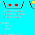Pages

Speed! - Number Sense - Simplifying Algebraic Expressions

Speed! is a fun game for learning multiplication, but playing Speed! also helps children develop a strong number sense. This is the third post in a series of posts - Speed! Number Sense - to explore different mathmatical concepts and how playing Speed! can facilitate understanding of these concepts.

The third concept is SIMPLIFYING ALGEBRAIC EXPRESSIONS. When children play Speed! they quickly become familiar with the numbers in the decks. Then the numbers become associated in their mind.   When a child who has played a lot of Speed! to learn the multiplication tables begins simplifying algebraic expressions, the number sense helps tremendously.

For example, each of the coefficients in this algebraic expression are in Four Speed. Four Speed contains the numbers 4, 8, 12, 16, 20, 24, 28, 32, 36 and 40.
A child given the task to simplify the expression would recognize that 4, 12 and 32 were cards in Four Speed. 4 was the first card, 12 was the third card, and 32 was the eighth card. Therefore the expression could be reduced to:
------------------------------------------------------------------------------------------------
The same is true for this algebraic expression, except all the numbers are in Six Speed.
It simplfies to:
Playing Speed! doesn't teach algebra, it is an activity that helps with multiplication and creates an excellent foundational number sense within children who play. It simply makes algebra a little easier.

1.In mathematics, an algebraic expression consists of variables, constants, operator signs (plus, minus, multiplication, division or exponentiation).

2.This comment has been removed by the author.

3.awesome, cards can be used for simplify an algebraic expression

Note: Only a member of this blog may post a comment.